# File Handling in Python

File Handling in Python

Now, we are going to have a look at a very interesting, and very useful concept, which is the concept of File Handling in Python. Here, we are going to discuss many file-related operations, like creating a file, writing some data to the file, reading data from the file, closing the file, or removing the file. We would also cover some methods. Here is an insight into what we are going to cover

• Why file handling?
• How to create a file (or how to open a file)?
• Writing data to the file
• write method
• writelines method
• Reading data from a file
• reading data line by line using for loop.
• The tell method in python
• The seek method in python
• The with keyword (use in file handling)

## File Handling in Python

Why file handling?

Well, when we write some programs, we work with some kind of data. If we are required to store the data somewhere in the program, we make use of variables. But if we want to have the data for some future use, we can store the data in the file, and access the file whenever needed. Now, since we are storing the data in the file, the data would be stored in some non–volatile storage. So, in order to work with files, we need to learn the concept of file handling. Also, at times, we might need to get the data from some file into our programs, and in such situations as well, we would require the concept of file handling. There are going to be different file operations that we are going to perform.

This is not just some concept that you need to learn, just because it is there in the curriculum, but it is really useful at times.

Now, that we are quite clear with what are we going to do throughout this concept, Let’s have a look at some different file operations that we are going to perform here. There are some basic operations that we usually carry out, which include –

• opening a file (if the file does not exist, we would create the file)
• Perform the operation (Read from the file, or write to the file)
• close the file.

This is just like what we usually do in our life when we are dealing with some files on our computer manually. Let’s say that I sent you a PDF file on your computer, and you want to read that PDF. So, you will simply open that file, read the data from the file, and then close the file. Also, you would do something similar while writing to some file (instead of a read operation, you are going to perform the write operation).

So, when we are clear about the operations that we are going to do, Let’s dive into understanding those different operations individually.

### How to create a file (or How to open a file)

Now, we are going to dive into the basics of file handling. Basically, when we are dealing with some file, we need to open the file, or if the file is not there, we need to create the file. So, here, we are going to explore what we can do to open a file or create a file.

In order to open a file, we simply have to make use of a built-in function in python, which is an open function. This function returns the file object, or the file handle, which we can work on. Also, we have to pass some arguments to the function, which simply means that we are just giving some data to the function so that it can do what it is supposed to do. So, now we are going to have a look at some of the different arguments that we provide for the open function. But even before that, have a look at a simple program, through which, we can get an idea about how we can write the open function.

As you can see, we have called the open function, and to the open function, we are passing some arguments as well. In both functions, the first argument that we are passing here is the pathname of the file. The second argument is the mode in which we are opening the file. There are different modes available when we are opening the file (we are going to mention the modes soon).

Have a look at the program and you can see that we have written the open method two times, wherein the first time, we are specifying only the filename, which means that the file would be opened from the current directory. When the second time we are writing the method, we are specifying the full path of the file. In both cases, the mode of the file is ‘w’, which means that the file would be opened in the write mode.

Remember that here, in the first open function, we are providing only the file name, so it is the relative path, and the operation would be done for the current directory. Since the mode is ‘w’, if the file does not exist in the given path, it would be created, and if the file already exists, then it is truncated, which simply means that the data from the file would not be retained.

Also, since the open function returns the file object, or handle, we have created a reference variable for that, which is f1 and f2 in the above case.

Now, Let’s have a look at some different modes, to open the file.

So, these are some different modes that we can give as an argument for the open method. Basically, if we are not giving the mode, it defaults to the read mode. We can also specify the encoding here, as an argument to the open function. Let’s have a look at the program below, which tries to demonstrate the same thing.

As you can see in the above program, we have specified the encoding as ‘utf-8’. If we do not specify the encoding, then the encoding used is platform-dependent.

So, coming back to the built-in open function, you can make use of this function, to open a file object, on which, we can do the necessary operations. As you can see in the previous program, the open function is returning the file object, for which, we have created a reference variable as well.

Let’s try the ‘x’ mode now, which is there for exclusive creation. But remember that it fails if the file already exists. Let’s have a look at the program now, which tries to demonstrate the same thing.

As you can see in the above program, we have given the mode ‘x’, which is for exclusive creation. If the file does not exist, then it would be created, and if it exists, then you would get an error saying that the file exists. You can try executing the program and observing the output as well.

So, now we are familiar with how we can open some files or create a file. There are some other arguments as well, which can be passed to the open function, other than the file path, and the mode, but we will use them as per the requirement. Also, if we are not providing any mode, in that situation, the default mode is the read mode. Note that, in some cases, if the file does not exist, it will be created, like in the write mode or the append mode. Let’s explore more file operations, since we are now familiar with how we can open the file or create the file.

### Writing data to the file

Now, we are quite familiar with how we can open some files or create some files. Now, we are going to have a look at how we can write some data to the file. This is just the write operation that we are going to perform now. Writing to the file simply means that we are writing some kind of data(string) into the file.

There are several methods, that we can use for writing some data to the file. Let’s have a look at them now. First of all, in order to be able to write into the file, we will have to have the file open in the write mode (or the append mode). So, we are already familiar with the fact, that in order to open the file in the read mode, we are required to use the ‘w’ mode in the open function, while we open the file basically. It is to be noted that if the file does not exist, it will be created in this case. Soon we are going to have a look at a simple program, which would demonstrate us creating the file for writing into the file. But even before that, Let’s have a look at one of the methods, with help of which, we are going to write to the file. Have a look at the below table, where you can find some methods for writing data to the file, and their description in brief.

Before we even move to explore these methods, we need to be aware that in order to write some data to the file, the file needs to be opened in write mode (‘W’), append mode (‘a’), or exclusive creation (‘X) mode. Also, if the file is in the write mode, and the file already exists in the given location, then all the previous data would be erased. Keeping all these things in mind, Let’s have a look at some methods for writing the data to the file now.

### Write method in python

In order to write some data to the file, we can make use of the write method. In the case of the text files, we need to pass in a string to the write method, and it writes it to the file and returns the number of characters written to the file. So, we are going to have a look at a simple program, where we need to open the file in the write mode, and then write some data using the write method, and then close the file. Let’s have a look at how we can do this.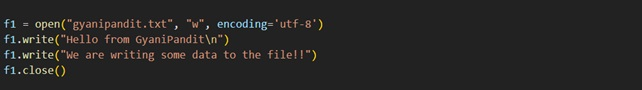As you can see in the above program, we opened the file in the ‘w’ mode, and then we are writing some data to the file, using the write method. We are also closing the file in the end, using the close method. This is basically a good practice, and we should close the file after we are done performing the required operation.

These are the contents of the file now –

Hello from GyaniPandit
We are writing some data to the file!!

As you can see, the required strings were written to the file. But, since we are using the write mode, the next time we run the same program, the previous data would be erased. You can try this, by just opening the file in the write mode, and trying to write some other data. You would find that the previous data was erased.

If we are required to retain the data, then we would have to open the file in the append mode(‘a’). Let’s have a look at a simple program, which demonstrates the same thing.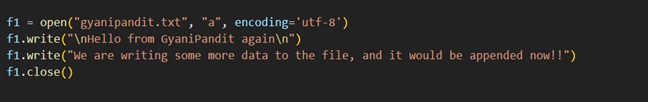As you can see in the above program, we have opened the file this time in the append mode, and when we are writing the data again, it would be appended, and the previous data would be retained. We had written some data previously to the same file, so due to the append mode, we have the data retained, and the new data would be appended. Let’s have a look at the data from the file now –

Hello from GyaniPandit
We are writing some data to the file!!
Hello from GyaniPandit again

We are writing some more data to the file, and it would be appended now!!

As you can see, the data was appended to the file, and the previous data was retained. So, according to the requirement, you can open the file in the write mode or the append mode. But the thing is that with the write mode, the content from the file would be erased if you open the file in the write mode, and that file already exists.

### Writelines method in Python

Now, Let’s have a look at the writelines method. Using this method, we can write the list of lines to the file. Let’s say that we have a list of lines that we want to add to the file. In such a situation, we can make use of the writelines method. Let’s have a look at the simple program, through which, we can understand the writelines method.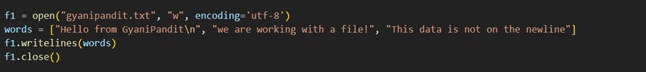As you can see in the above program, we have opened a file in the write mode, and if the file already exists, all the data from the file would be erased, and then we have a list, which contains some strings, and we want to add these lines to the file. In such a situation, we have made use of the writelines method. Let’s have a look at the data from the file now.

Hello from GyaniPandit
we are working with a file! This data is not on the newline

As you can see in the output, we have the words added to the file, which were in the given list.

So, as, and when required, we can make use of the writelines method, to write the list of lines to the file. Again, here as well, since we are opening the file in the write mode, if the file exists already, then the previous data would be erased. Again, if you want to overcome this, you can open the file in the append mode. You can try the program for the same and try to observe the output as well.

So, this was about writing data to the text file. We can make use of the given write method, and the writelines method, on the basis of our requirements. Remember that we can write some data to the file when the file is opened in the write mode, the append mode, or the exclusive creation (‘X) mode.

### Reading from the file in Python

Now, we are going to learn to read from the file. This is also a simple and usual operation that we would perform on the file. In order to read from the file, we need to open the file in read mode ( ‘r’ ). In order to read from the file, there are various things that we can do here, and we are going to explore them now.

Now, we are going to explore some methods, related to reading from a file.

Now, we are going to explore these methods one by one, and also, we are going to learn how to read data from the file, using the for a loop. But even before that, understand that in order to read data from the file, we need to open the file in read mode.

Now, we are going to learn about the read method. As the name of the method says, using this method, we are going to read some data from the file. We can provide a size argument, and if we provide the size argument, then it reads the size number of data. If the size is not provided or is negative, then the entire contents are read and returned. Let’s have a look at a program, which demonstrates the same thing.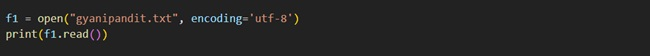As you can see in the above program, we have used the open function to open the file. Basically, since we are not providing any mode, it defaults to the read mode. Also, we are calling the read method, to read the data from the file. Since we have not specified the size of the data that we want to read, it would read the entire data and return it. We are printing the data. Let’s have a look at the output from the above program.

Hello from GyaniPandit
We are working on the files now!!
This is some more data in the file.

As you can see, we could read all the contents of the file. Now, Let’s say that if we specify the size argument with the read method, it reads at most size characters. Let’s have a look at another program, in which we would try to give the size argument to the read method.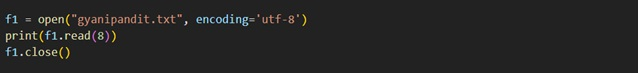As you can see in the above program, we have specified the size argument this time, and as the output, the read method reads at most size characters. Let’s have a look at the output now –

Hello fr

As you can see, we could read 8 characters from the text file. So, this way, we can simply use the read method, to read the data from the file. Remember that we can specify the size as an argument, and if we are providing the size argument, at most size characters are read. If the size argument is negative, or not given, then the entire data is read and returned.

So, as per our requirement, we can make use of the read method for reading data from the file. Remember that we can give the size as an argument.

Now, we are going to learn about the readline method. As the name of the method suggests, using this method, we can read a single line from the file. Let’s have a look at the example, which demonstrates the same thing about the readline method.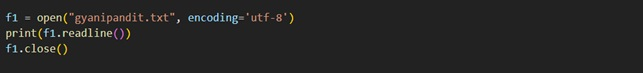As you can see in the output, we have used the open function to open the file, and then we have called the readline method. With this method, we would read the single line from the file. Let’s have a look at the output now.

Hello from GyaniPandit

As you can see in the output, we got one line from the file. You can call the readline method multiple times. Let’s have a look at a simple program again, which demonstrates the same thing.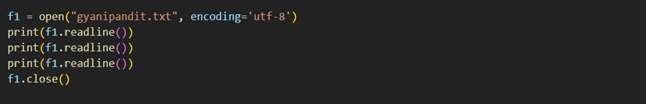As you can see in the above program, we have the same file, and we are calling the readline method multiple times. Let’s have a look at the output from the above program.

Hello from GyaniPandit
We are working on the files now!!
This is some more data in the file.

As you can see, we got the required output. So, with the readline method, we can simply read a single line from the file. So, as and when required, we can make use of the readline method, to read a line from the file.

Now, we are going to learn about the readlines method. We can make use of the readlines method, when we want to read all the lines from the file, in a list. Let’s have a look at a simple program, which demonstrates the same thing.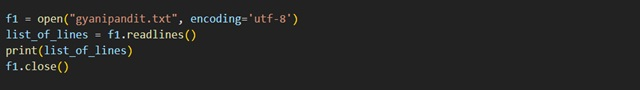As you can see in the above program, we have used the open function, and then we have called the readlines method. The readlines method returns the list of lines from the file. So, we are assigned the list to the variable list_of_lines, and then we are printing the list. Now, Let’s have a look at the output of the above program.

[‘Hello from GyaniPandit\n’, ‘We are working with the files now!!\n’, ‘This is some more data in the file. \n’]

As you can see, we have got the list, with all the lines from the file. So, whenever we are required to get the list of lines from the file, we can make use of the readlines method. Alternatively, we can also use the list constructor, to get the list of lines from the file. Let’s have a look at that too.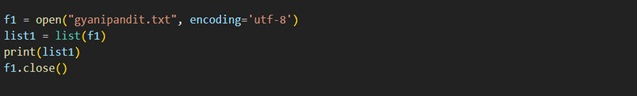As you can see in the above program, we are doing the same thing, but this time, we are making use of a list constructor. You can refer to the above program and understand how did we do that. We are then printing the list of lines from the file. So, these were some things that we can do, if we want to read all the lines from the file into a list.

### Reading from a file using for loop in Python

We can read the file line by line using the for loop as well. It is very fast and efficient as well. So, Let’s have a look at a simple program, which demonstrates to us about reading data from the file, line by line, using the for a loop.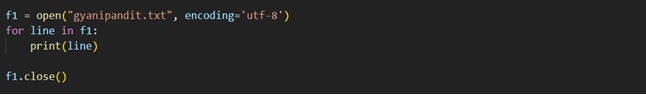As you can see in the above program, we have used a for loop and using that, we are reading the data, line by line. So, we can make use of them for a loop too, to read the data from the file, line by line.

### Tell method in python

Now, we are going to learn about the tell method in relation to file handling. The tell method returns an integer, which specifies the current position of the file object in the file. Let’s have a look at a simple example, which demonstrates the tell method.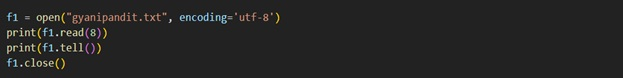As you can see in the above program, we opened the file using the open function, and then we read 8 characters from the file, and then we called the tell method. The tell method returns an integer, which specifies the current position of the file object in the file.

So, as and when required, we can make use of the tell method in our python program. Remember that the tell method is going to return an integer, which specifies the current position of the file object in the file.

### Seek Method in Python

Now, we are going to learn about the seek method in relation to file handling. Well, using the seek method, we can change the file object’s position. We can get the file object’s current position using the tell method. So, whenever we need to change the file object’s position, we can make use of the seek method.  Let’s have a look at a simple program, which demonstrates the seek method.As you can see in the above program, we have read a line using the readline method. Then we are getting the current position of the file object using the tell method. Then we are calling the seek method, and to the seek method, we are giving the argument, which is the offset (we will understand the offset in greater detail soon), but for now, we can consider that with the seek method, we took the file object position, back to 0. After that, we are again getting the current position of the file object, and we find that this time, it is set to 0.

So, using the seek method, we can change the position of the file object. Now, Let’s explore the seek method in greater depth.

To the seek method, we need to provide some arguments, like offset, and whence.  Basically, the new position is computed by adding the offset to a reference point. The reference point is selected by the whence argument. We can have 0 or 1 or 2 as a value for the whence argument. Well, 0 measures from the beginning of the file, while value 1 uses the current file position, and value 2 uses the end of the file as a reference point. If we omit this whence argument, it defaults to 0, which means that it uses the beginning of the file as a reference point.

So, as and when required, we can make use of the seek method in our programs. Using the seek method, we can change the position of the file object’s position. We can specify the offset and the whence argument. The new position is determined by adding the offset to the reference point, and the reference point is selected by the whence argument.

### With keyword (use in File Handling in Python)

Now, we are going to learn about the keyword in python. Basically, the keyword in python is used for resource management and exception handling. You would most likely see the keyword being used when working with the file stream. It simplifies the management of resources like file streams.

You might be familiar with the thing, that when we are done with some file operations, we should close the file to release the resources. Let’s have a look at a simple program, where we are going to work with files, and we are going to surround them with the try-catch block.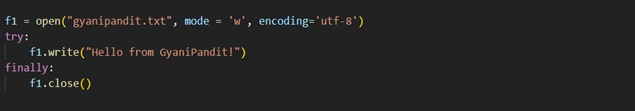As you can see in the above program, we are doing a simple file operation. We are opening the file, then we are reading from the file, and then we are closing the file. But now, Let’s try doing the same thing using the keyword.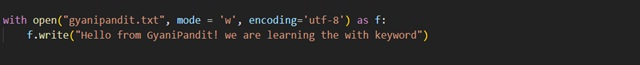As you can see here, there is no need to call the close method, when we are using the keyword. The with statement ensures the proper acquisition and release of the resources. Basically, this is done because we need to make sure that the resources that were allocated are released. So, the with statement avoids any bugs and leaks ensuring the proper release of the resource.

So, with this, we have explored a lot, about the Python programming language. As you can see, the language is very easy to understand and implement. You can further learn, explore and implement different concepts, and practice the concepts that you are already familiar with, so that you become good at those concepts, and be comfortable while using these concepts. All the best and keep learning!